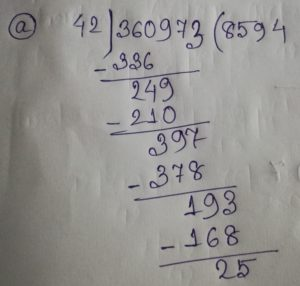# S. K. Gupta Anubhuti Gangal Class 5 Math First Chapter Looking Back Exercise 4

## S. K. Gupta Anubhuti Gangal Class 5 Math First Chapter Looking Back Exercise 4

EXERCISE 4

(1) Divide:

(a) 360973 ÷ 42(b) 196997 ÷ 50

(c) 274516 ÷ 63

(d) 258277 ÷ 49(2) Divide and check:

(a) 835 ÷ 39

(b) 3958 ÷ 57

(c) 30059 ÷ 48(3) About 13,200 babies are born in the world every hour. About how many are born in a minute?

Solution: Here, 1 hour = 60 minutes

So, 13200 ÷ 60 = 220

Hence, 220 babies are born in a minute.

(4) Tell which of the following meaningless operations are:

(a) 0 ÷ 5(c) 21 ÷ (7 – 7)

(d) 8 ÷ 0

(e) (9 – 9) ÷ 32

(5) A girl knits 718 stitches. If there are 25 stitches in each row of knitting an hour. How many complete rows have been knitted?

Solution:Hence, 28 rows have been knitted with 700 stitches and 18 stitches more

(6) A rocket travelled at a speed of 29760 kilometers an hour. How many kilometers would the rocket traveled in one minute at this speed?

Solution: Here, 1 hour = 60 minutes

29760 ÷ 60 = 496 kilometers

Hence, the required distance is 496 kilometers.

(7) The product of two numbers is 71424. If one number is 93, what is the other number?

Solution: The other number = 71424 ÷ 93 = 768

Hence, the required number is 768.

(8) In a garden there are 4,891 trees in 67 rows. If each row has the same number of trees, how many trees are there in one row?

Solution: 4891 ÷ 67 = 73 trees

Hence, there are 73 trees in a row.Updated: May 31, 2022 — 12:14 pm